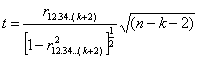# Statistics Assignment Help With Testing The Significance

## 12.2.7 Testing the significance of an observed partial correlation coefficient.

Let r12.34….(k+2) be the partial correlation coefficient of order k, observed in a sample of size n from a multivariate normal population. Prof. R.A.Fisher proved that under the null hypothesis H0: ρ12.34….(k+2) = 0 that is the population correlation coefficient is zero, the sampling distribution of r12.34….(k+2) is same as that of r12 under the null hypothesis ρ12 = 0, with sample size n reduced by k. hence the test statistic for H0 becomesWhich follows Student’s t – distribution with (n-k-2) degrees of freedom.